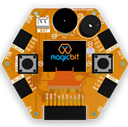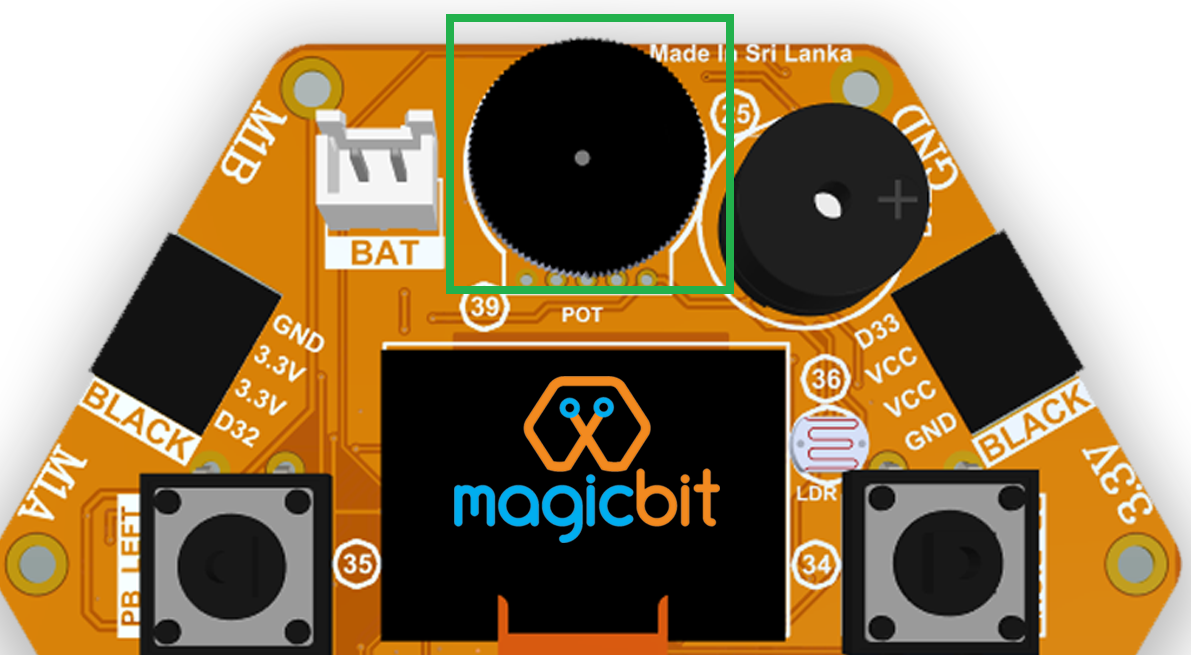#### Components Required#### Introduction

In this example, you are learning to read an analog sensor.

Learning Outcomes

From this example, you’ll get an understanding about,

#### Theory

In the real world most of the signals we encounter are analog signals (temperature, air pressure, velocity), they are continuous. But computers work in the digital domain, to interact between the worlds, representing an analog signal in the digital domain is important. (to read more about analog to digital conversion, follow this link)

#### Methodology

For this example, we use the potentiometer on the Magicbit board, which is connected to pin, D39. It generates a voltage between 0 and 3.3V according to the angle of the potentiometer.We read the analog signal and store it in an int type variable(0v= 0 analog value, 3.3v = 4096 analog value), sensorValue, later, we use this value to light up the red LED(D27) if the analog value exceeds 2048.

#### Code

```from machine import Pin,ADC
LED=Pin(16,Pin.OUT)
while True:
if sensorValue>2048:
LED.off()
else:
LED.on()```